### IMO Shortlist 2018 problem A4

Kvaliteta:
Avg: 0,0
Težina:
Avg: 7,0

Let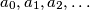$a_0,a_1,a_2,\dots$ be a sequence of real numbers such that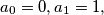$a_0=0, a_1=1,$ and for every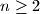$n\geq 2$ there exists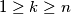$1\geq k \geq n$ satisfying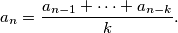Find the maximum possible value of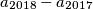$a_{2018}-a_{2017}$.

Izvor: https://www.imo-official.org/problems/IMO2018SL.pdf